# Connecting Thevenin sources/Norton sources.

#### Xenon02

Joined Feb 24, 2021
364
Hello !

I've got a question.
I know it is stupid but I'm asking it theoretically.

For example can I calculate from this circuit : AC = 5V (peak), DC = 1V, R1 = R2 = 1k

Can I calculate Thevenin source DC and Thevenin source AC, Rw and Zw and connect them together to calculate voltage on R2 ?
Like here ? Because if I had R2 not as linear resistor but as nonlinear resistor then my teacher said I can't connect Thevenin DC and AC like below, so I thought if I can do it for linear components.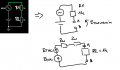EDIT:

I'm asking this question because I tried to do it for Non linear resistance and my teacher said I can't connect Thevenin source AC and DC.
So I don't know how it works. Can I connect them this way? (I know this doesn't make sense and I can do it differently, but I want to know).
This way I mean calculating Thevenin source DC and Thevenin source AC and connect them in one circuit ? I've heard that I can do it and I've heard that I can't do it. But I don't know what is the truth.

Last edited:

#### WBahn

Joined Mar 31, 2012
29,163
As soon as you start talking about nonlinear elements, you have to be extremely careful because the very definition of Thevenin and Norton equivalency is based on linear systems.

If you leave the nonlinear element out of the equivalent circuit portion, then you are good. This is commonly how nonlinear circuits are analyzed -- you reduce the linear portion of it to simple equivalents connected to the nonlinear component, which allows you to do things like a load-line analysis.

In your diagram, what is Rw and Zw in terms of R1. There's no way to tell if you are doing it correctly or not.

For your example circuit, the Thevenin equivalent circuit as seen by R2 is the DC source in series with the AC source in series with R1. In other words, you already have the Thevenin equivalent right there.

#### Xenon02

Joined Feb 24, 2021
364
As soon as you start talking about nonlinear elements, you have to be extremely careful because the very definition of Thevenin and Norton equivalency is based on linear systems.

If you leave the nonlinear element out of the equivalent circuit portion, then you are good. This is commonly how nonlinear circuits are analyzed -- you reduce the linear portion of it to simple equivalents connected to the nonlinear component, which allows you to do things like a load-line analysis.

In your diagram, what is Rw and Zw in terms of R1. There's no way to tell if you are doing it correctly or not.

For your example circuit, the Thevenin equivalent circuit as seen by R2 is the DC source in series with the AC source in series with R1. In other words, you already have the Thevenin equivalent right there.
I mean more likelely that can I calculate Theremin equivalent for AC and DC using superposition and add them in one Circuit?
Because for nonlinear resistor I couldn't because my teacher said that they are 2 different circuits (the nonlinear one).
So I could only use thevenin equivelent for nonlinear Elements seperately which mean Thevenin DC equivelant seperately and Thevenin AC equivelant seperately from DC Thevenin equivelant.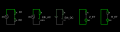Adding all 4 parts in one circuit :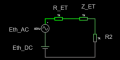Also I have to use superposition here to calculate R2 (So for DC I had to take out Z_TH and Eth_AC, for AC superposition I had to take R_TH and Eth_DC) right ? If Z_TH was a complex number and not a real like here then I had to make a superposition to calculate voltage on R2 I guess ?

Also what If I was calculating Thevenin equivelants (AC and DC) for not R2 but for C1 (capacitor). Could I also do it?
What do I mean is using superposition for AC and DC. Calculate DC Thevenin and AC Thevenin, calculating R_Th and Z_Th for DC and AC. And connect all 4 parts in series and connect also in series C1 ?

But I don't Understand why.
Why for linear I can add DC and AC equivelants of Thevenin in one Circuit.

Rw and Zw are like Rth and Zth. Those equivelants for DC Thevenin load Rth and AC Zth.

For example of nonlinear circuit (I know how to solve it but) :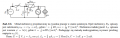For more clarification I have to calculate un(t) = AC+DC. Ln is nonlinear using equation b*i|i| and b is also equal 1/6 mWb.
Rn is equal Un =r*i_n+a*i_n^3 r = 1/2k, a = 1/18.

I am not looking for answear what is the Un here. I am looking for answear why I can calculate here Norton equivelant DC and Norton equivelant AC and add them in one circuit like here :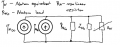why I can't do it for nonlinear but I can do it for linear ?

Last edited:

#### WBahn

Joined Mar 31, 2012
29,163
You are making a mistake from the beginning when you are trying to use superposition. Superposition is used to find a voltage or a current, not an impedance.

Consider the method used to find Z_th. Turn off all the sources and determine the impedance as seen by the load. It doesn't matter how many sources there are. It doesn't matter whether they are DC or AC. You find a single Z_th and that is the Z_th for your Thevenin equivalent circuit. Now you turn to finding the Thevenin equivalent voltage and one option is to use superposition. But you only add the results of that in order to find V_th. It has NO effect on Z_th.

Consider the case of 100 1.5 V series-connected batteries in series with a 1 kΩ resistor. What is the Thevenin equivalent for that? By inspection you should be able to see that Vth = 150 V and Rth = 1 kΩ since you can just combine the 100 batteries into a single equivalent battery and be done. But using your method, you would say that you have V_th = 150 V and R_th = 100 kΩ.

#### Xenon02

Joined Feb 24, 2021
364
Consider the method used to find Z_th. Turn off all the sources and determine the impedance as seen by the load. It doesn't matter how many sources there are. It doesn't matter whether they are DC or AC. You find a single Z_th and that is the Z_th for your Thevenin equivalent circuit. Now you turn to finding the Thevenin equivalent voltage and one option is to use superposition. But you only add the results of that in order to find V_th. It has NO effect on Z_th.
Z_th has effect whether it is AC or DC.

For example here :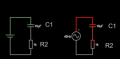On the left is DC circuit on the right AC circuit let's calculate voltage on R2 for both cases.
For the left one Thevenin voltage is 1V, but Z_th for DC is infinite because if I turn off the DCvoltage I have only capacitor, capcitor is for DC a open circuit.

So The Thevenin equivelant is 1V and Z_th = infinite. So R2 get's 0 V.

For AC it is different. I get 5V peak Thevenin Voltage AC, and the Z_Th is equal to capacitor impedance. Because for AC I use imaginable value.

And now R2 gets a voltage.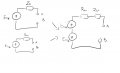Last edited:

#### WBahn

Joined Mar 31, 2012
29,163
If you are going to use reactive components in a multi-frequency system, you have to use symbolic impedances because the impedance is frequency-sensitive.

It looks like you R2 is your load, so Z_th is -j/(wC). That is the Thevenin impedance.

In your approach, you are putting an infinite impedance in series with a another impedance. The result is an infinite impedance and no current will flow.

#### Xenon02

Joined Feb 24, 2021
364
It looks like you R2 is your load, so Z_th is -j/(wC). That is the Thevenin impedance.

In your approach, you are putting an infinite impedance in series with a another impedance. The result is an infinite impedance and no current will flow.
Yes so Z_th depends on if it's AC or DC.
For AC The capacitor is a open circuit so Z_th dc = infinite, same goes with AC the impedance is now not infinite but -j/(wC).

So Z_th depends on source what I have. For AC It is different and for DC it is different. Like I have shown in the picture.

So connecting AC Thevenin and DC thevenin + connecting Z_ThDC and Z_ThAC in series will give me a wrong result.

So I can't just turn off both sources and calculate the Z_Th. They are different depending on the source.

I will then have something like this: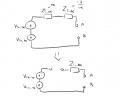#### WBahn

Joined Mar 31, 2012
29,163
Again, the Thevenin impedance is -j/(wC). Period. It doesn't matter whether it is a DC source or an AC source or twenty different AC sources all at different frequencies. The single Thevenin impedance is -j/(wC).

When you want to find the total voltage across the load, because you have sources at different frequencies, you use superposition.

Remember, DC is just a special case of AC in which the frequency happens to be zero.

#### Xenon02

Joined Feb 24, 2021
364
Again, the Thevenin impedance is -j/(wC). Period. It doesn't matter whether it is a DC source or an AC source or twenty different AC sources all at different frequencies. The single Thevenin impedance is -j/(wC).

When you want to find the total voltage across the load, because you have sources at different frequencies, you use superposition.

Remember, DC is just a special case of AC in which the frequency happens to be zero.
So why when I had 2 circuits and I tried to calculate for them Thevenin impedance it was different for AC and DC.

AC :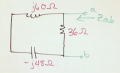DC doesn't have imaginary value.
At least that's how we do it in school if we want to calculate E_th for AC circuit or for DC circuit.

Also let's say it works this way. Then why can't I do it for nonlinear resistors ? Like in the example above I gave.

Last edited:

#### WBahn

Joined Mar 31, 2012
29,163
You are trying to make a Thevenin circuit that has multiple impedances, one for each source, in series. That is NOT the same thing as having a single impedance whose value is a function of frequency.

DC has an imaginary component, that component happens to have the value zero.

As I said before, if you have a circuit that has nonlinear elements, you can only replace those portions of the circuit that are linear with the Thevenin equivalents for portions. The very notion of Thevenin equivalency relies on superposition, which in turn is only valid for linear systems.

#### Xenon02

Joined Feb 24, 2021
364
Okey so in my example I gave if that inductor was linear then i could replace everything with VTh AC DC and Zth and add to A B nodes non linear resistor?

Also that's wierd that I can calculate multiple sources but not multiple impedances.

#### Xenon02

Joined Feb 24, 2021
364
For this example I gave here :I tried to calculate Norton DC.

So because this is DC then Ln which is nonlinear is still short circuited capacitor is an open circuit :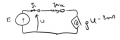But the problem is for Norton impedance because there is still non linear inductance. But if it was linear inductance then I can calculate Norton AC and Norton impedance and connect 3 parts in parallel ?

#### WBahn

Joined Mar 31, 2012
29,163
Okey so in my example I gave if that inductor was linear then i could replace everything with VTh AC DC and Zth and add to A B nodes non linear resistor?

Also that's wierd that I can calculate multiple sources but not multiple impedances.
Not weird at all. Go back and study what it means for a system to be linear and what superposition means.

Consider if you have two voltage sources in series, v1(t) and v2(t), connected to a an impedance R.

The current through resistor is i(t) = (v1(t) + v2(t))/R = v1(t)/R + v2(t)/R

This true because the resistance is linear, and because this is true, I can write this as

i(t) = i1(t) + i2(t)

where

i1(t) = v1(t)/R (i.e., the current through R when v2 is set to zero)
and
i2(t) = v2(t)/R (i.e., the current through R when v1 is set to zero)

This would not work if R was not linear. Try it with a nonlinear R, for instance an R were i(t) = k*v^2(t) where k = 10 mA/V^2

The Thevenin equivalent for this just has one R in it -- it does not have a separate R for each source.

#### Xenon02

Joined Feb 24, 2021
364
So what do you think about my calculation for non linear resistor as a load ? But the problem is with Ln - non linear inductance and I can't calculate Thevenin impedance. But this is ideal norton source because if I want to calculate thevenin source then I can't, becasue contrary to circuit theory.
But I don't know. It's hard to see it in non linear example. I don't know if I can use Norton/Thevenin or not. For this task I uploaded above with Rn and Ln -non linear components.They said I could use Norton equivalent but here I have non linear components

So when I could calculate the Norton source like I did above, then Norton impedance is (infinite), so I can calculate Norton source AC and connect AC and DC Norton source and Z_N parallel with non linear resistor (non linear resistor is a load) ?

Something like this? :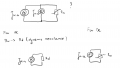Last edited:

#### Xenon02

Joined Feb 24, 2021
364

#### WBahn

Joined Mar 31, 2012
29,163
If you are including a nonlinear component as part of the Thevenin/Norton equivalent, then you are going to have problems. The nonlinear components need to be part of the load.

#### Xenon02

Joined Feb 24, 2021
364
In this example and my calculation can it work?
Because I can't calculate Thevenin DC I could only calculate Norton DC so I thought that this is ideal Norton source where Norton impedance was equal infinite? And so impedance doesn't depend on AC and DC so Norton impedance is always infinite? I don't know of I made a right calculation. Im a bit confused.

But calculating it normaly would be a problem maybe i don't know. Im trying the option that this task gave me which is Norton Thevenin and I wanted to Connect Norton AC and DC and the Norton load for non linear Resistance I didn't know if its possible

Last edited:

#### WBahn

Joined Mar 31, 2012
29,163
In order to evaluate your calculations, you need to translate the problem that you gave into English and then show your work step by step. I'm not going to spend a bunch of time guessing.

#### Xenon02

Joined Feb 24, 2021
364
I think I did translate the task.
The task was to calculate Voltage on nonlinear Resistance.
There were 2 ways to calculate it. Using small Signal method or Norton/Thevenin.

I also translate the data for Rn and Ln which are the non linear Resistance and non linear inductance.

In the picture from post 12 I used super position and replaced RN to short Circuit to get the Norton DC.

Replacing the short Circuit into Open Circuit will result in circuit Theory error.
And I can't calculate the Thevenin DC.

So I came up with idea that it must be ideal source. Norton DC, Norton impedance is infinite, but I don't know if It is also infinite for AC Norton source.

So I don't know if I can Connect AC Norton, DC Norton and infinite impedance Parallel with non linear impedance. Knowing that in Norton there is non linear inductor.

Here are the pictures :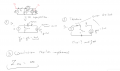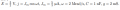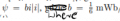- for non linear inductance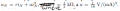= for non linear resistance.

As I mentioned I somehow calculated Norton equivelant DC but I couldn't calculate Thevenin equivelant DC so I went with conclusion that Norton impedance is infinite for DC. But you mentioned also that the sources doesn't matter so for AC it is also infinite impedance ?
So I don't know yet how to calculate Norton equivelant AC. But the matter here is if I calculate Norton equivelant AC and DC (with the nonlinear component in them) then I can add them (I mean AC Norton equivelant and DC Norton equivelant and Norton impedance which is infinite) in parallel with non linear resistance even thought the equivelants and norton resistance consist nonlinear component?

Last edited: